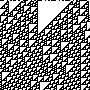# Representing Graphs

Example 1:

1 First node2 Second node#1 2 Edge between the two

Example 2:

0 "Door" type=object1 "Open" type=state2 "Closed" type=state3 "Opening" type=process4 "Closing" type=process#0 1 "has state"0 2 "has state"3 0 "changes"3 1 "to state"4 0 "changes"4 2 "to state"

Example 3:

[tgf engine:graphapi_pngPRDACCTSTDEVgit#PRD PRD dbupdate, migrate, backup, restorePRD ACC syncACC git pullDEV git commit, pushTST git pullPRD TST syncTST PRD syncPRD git pull]

## 2. Newick format

Example 1:

(,,(,));                               no nodes are named(A,B,(C,D));                           leaf nodes are named(A,B,(C,D)E)F;                         all nodes are named(:0.1,:0.2,(:0.3,:0.4):0.5);           all but root node have a distance to parent(:0.1,:0.2,(:0.3,:0.4):0.5):0.0;       all have a distance to parent(A:0.1,B:0.2,(C:0.3,D:0.4):0.5);       distances and leaf names (popular)(A:0.1,B:0.2,(C:0.3,D:0.4)E:0.5)F;     distances and all names((B:0.2,(C:0.3,D:0.4)E:0.5)F:0.1)A;    a tree rooted on a leaf node (rare)

Example 2:

(((a,c),b),((d,e),(f,g)))

Example 3:

(A(B(C,D(E))F(G,H)));

Example 1:

## 5. DGML

<?xml version='1.0' encoding='utf-8'?><DirectedGraph xmlns="http://schemas.microsoft.com/vs/2009/dgml">  <Nodes>    <Node Id="a" Label="a" Size="10" />    <Node Id="b" Background="#FF008080" Label="b" />    <Node Id="c" Label="c" Start="2010-06-10" />  </Nodes>  <Links>    <Link Source="a" Target="b" />    <Link Source="a" Target="c" />  </Links>  <Properties>    <Property Id="Background" Label="Background" DataType="Brush" />    <Property Id="Label" Label="Label" DataType="String" />    <Property Id="Size" DataType="String" />    <Property Id="Start" DataType="DateTime" />  </Properties></DirectedGraph>

## 6. DOT / Graphviz

Example 1:

graph graphname {     a -- b -- c;     b -- d; }
digraph g {	node [shape=plaintext];	A1 -> B1;	A2 -> B2;	A3 -> B3;		A1 -> A2 [label=f];	A2 -> A3 [label=g];	B2 -> B3 [label="g'"];	B1 -> B3 [label="(g o f)'" tailport=s headport=s];	{ rank=same; A1 A2 A3 }	{ rank=same; B1 B2 B3 } }

Example 2:

Example 3:

## 7. TikZ

Example 1:

% Drawing a graph using the PG 3.0 graphdrawing library% Author: Mark Wibrow\documentclass[tikz,border=10pt]{standalone}\usetikzlibrary{graphdrawing}\usetikzlibrary{graphs}\usegdlibrary{trees}\begin{document}\begin{tikzpicture}[>=stealth, every node/.style={circle, draw, minimum size=0.75cm}]\graph [tree layout, grow=down, fresh nodes, level distance=0.5in, sibling distance=0.5in]    {        4 -> {           3 -> { 1 -> { 5, " " }, 2,2 },          3 -> { 1, 2, 2 },          3 -> { 1, 2, 2 }        }     };\end{tikzpicture}\end{document}
% Author: Till Tantau% Source: The PGF/TikZ manual\documentclass{article}\usepackage{pgf}\usepackage{tikz}\usetikzlibrary{arrows,automata}\usepackage[latin1]{inputenc}\begin{document}\begin{tikzpicture}[->,>=stealth',shorten >=1pt,auto,node distance=2.8cm,                    semithick]  \tikzstyle{every state}=[fill=red,draw=none,text=white]  \node[initial,state] (A)                    {$q_a$};  \node[state]         (B) [above right of=A] {$q_b$};  \node[state]         (D) [below right of=A] {$q_d$};  \node[state]         (C) [below right of=B] {$q_c$};  \node[state]         (E) [below of=D]       {$q_e$};  \path (A) edge              node {0,1,L} (B)            edge              node {1,1,R} (C)        (B) edge [loop above] node {1,1,L} (B)            edge              node {0,1,L} (C)        (C) edge              node {0,1,L} (D)            edge [bend left]  node {1,0,R} (E)        (D) edge [loop below] node {1,1,R} (D)            edge              node {0,1,R} (A)        (E) edge [bend left]  node {1,0,R} (A);\end{tikzpicture}\end{document}

Adjacency Matrix, Edge List, Neighbor List, Paths

## 9. Expressions

====

Misc sources:

by the author.

--

--

--

Love podcasts or audiobooks? Learn on the go with our new app.## Semiconductor and People (part 2.)## Creation of a digital twin of the Kaluga trolleybus## Visualizing Poetry using DALL-E## Knight’s Yarn